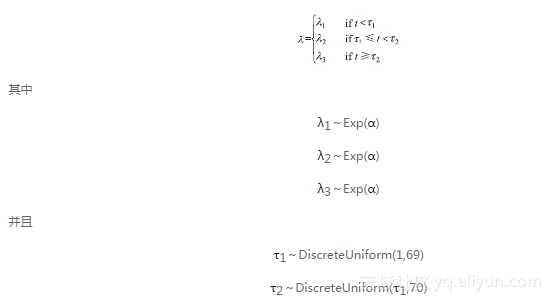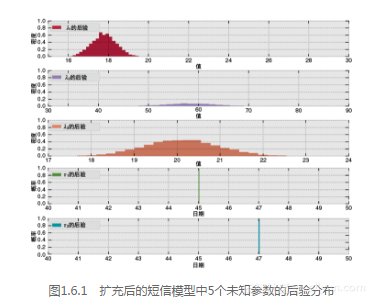#《贝叶斯方法：概率编程与贝叶斯推断》一1.6　补充说明

0
0
01. 云栖社区>
2. 博客>
3. 正文

## 《贝叶斯方法：概率编程与贝叶斯推断》一1.6　补充说明

### 1.6.1　从统计学上确定两个λ值是否真的不一样

print (lambda_1_samples < lambda_2_samples)
# Boolean array: True if lambda_1 is less than lambda_2.

[Output]:

[ True True True True ..., True True True True]

# How often does this happen?
print (lambda_1_samples < lambda_2_samples).sum()

# How many samples are there?
print lambda_1_samples.shape

[Output]:

29994
30000

# The ratio is the probability. Or, we can just use .mean:
print (lambda_1_samples < lambda_2_samples).mean()

[Output]:

0.9998

# The vector abs(lambda_1_samples - lambda_2_samples) > 1 is a boolean,
# True if the values are more than 1 apart, False otherwise.
# How often does this happen? Use .mean()
for d in [1,2,5,10]:
v = (abs(lambda_1_samples - lambda_2_samples) >= d).mean()
print "What is the probability the difference is larger than %d\
? %.2f"%(d,v)

[Output]:

What is the probability the difference is larger than 1? 1.00
What is the probability the difference is larger than 2? 1.00
What is the probability the difference is larger than 5? 0.49
What is the probability the difference is larger than 10? 0.00

### 1.6.2　扩充至两个转折点lambda_1 = pm.Exponential("lambda_1", alpha)
lambda_2 = pm.Exponential("lambda_2", alpha)
lambda_3 = pm.Exponential("lambda_3", alpha)

tau_1 = pm.DiscreteUniform("tau_1", lower=0, upper=n_count_data-1)
tau_2 = pm.DiscreteUniform("tau_2", lower=tau_1, upper=n_count_data)

@pm.deterministic
def lambda_(tau_1=tau_1, tau_2=tau_2,
lamb-da_1=lambda_1, lambda_2=lambda_2, lambda_3 = lambda_3):
out = np.zeros(n_count_data) # number of data points
out[:tau_1] = lambda_1 # lambda before tau is lambda_1
out[tau_1:tau_2] = lambda_2
out[tau_2:] = lambda_3    # lambda after (and including) tau
# is lambda_2
return out
observa-tion = pm.Poisson("obs", lambda_, value=count_data,observed=True)
mod-el = pm.Model([observation, lambda_1, lambda_2, lambda_3, tau_1,
tau_2])
mcmc = pm.MCMC(model)
mcmc.sample(40000, 10000)+ 关注